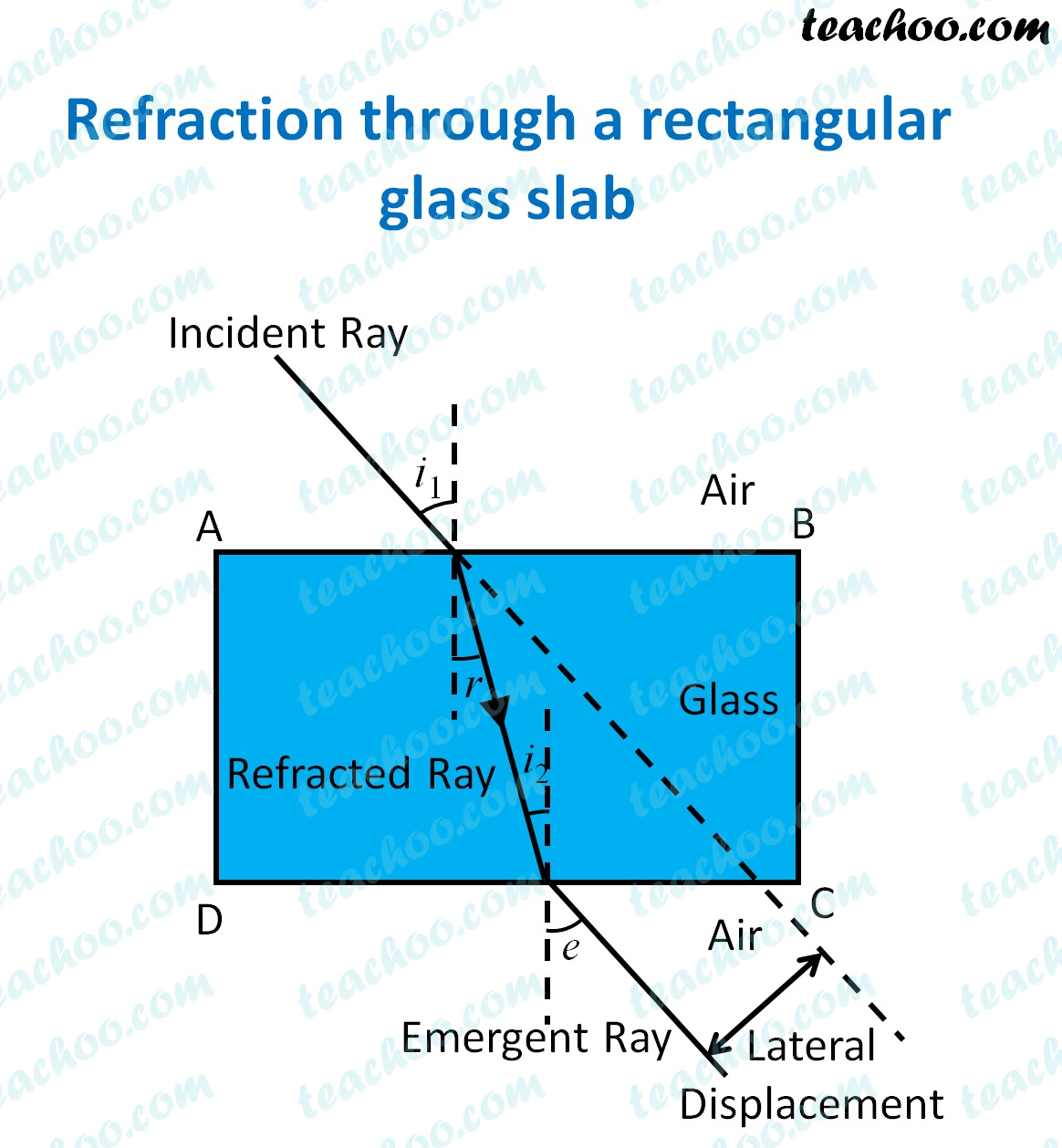MCQ from Past Year Papers

Class 10
Chapter 10 Class 10 - Light - Reflection and Refraction (Term 1)

## (d) ∠i is less than ∠e, but nearly equal to ∠r• When light enters from air to glass, it bends towards the normal
• Hence, angle of refraction is less than angle of incidence
• When light leaves the surface of glass to air
• It bends away from the normal
• This, angle of emergence is almost equal to angle of incidence

So, the correct answer is (a) - ∠i is more than ∠r, but nearly equal to ∠e